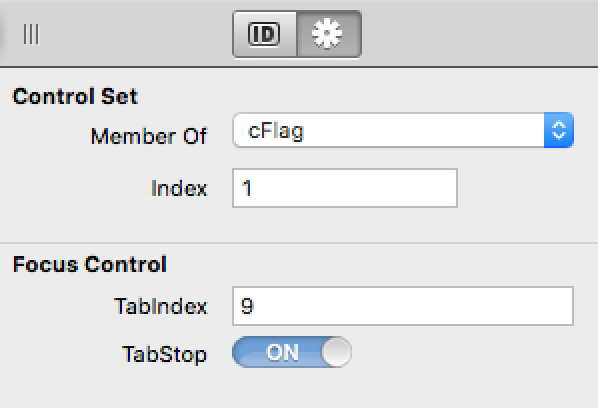# How do I get the Index value of a Control that is in a Control Set?In fact, How do I know by code that this cFlag Control is a member of a Control Set ?

Then, how do I get its Index ?

These are the information shown in the Control Set of the above screen shot.

Nota: Focus Control values can be get using Canvas1.TabIndex and Canvas1.TabStop.

You can check the Index property of the control.

``````var controlIndex as Integer = me.Index
``````

Thank you Anthony, but what about outside of the Control Set ?

Say in a PushButton or in a Method (somethng that scan a window contents).

I’m not sure I understand what you’re after. Can you explain what you’re trying to achieve?

If you want to iterate over controls in a ControlSet, here’s a thread that may help you:

Here’s a slightly more succinct method I just worked up:

``````Private Function ControlSetLastIndex(c as RectControl) As Integer
var lastIndex as Integer = -1
for each r as RectControl in c.TrueWindow.Controls
if r.Name = c.Name then
lastIndex = lastIndex + 1
end if
next r

Return lastIndex
End Function
``````

Called as:

``````var lastIndex as Integer = ControlSetLastIndex( PushButton1(0) )
``````

Note that you must pass it a valid instance from the ControlSet.

If you want it as an extension of RectControl, place in a module:

``````Public Function ControlSetLastIndex(extends c as RectControl) As Integer
var lastIndex as Integer = -1
for each r as RectControl in c.TrueWindow.Controls
if r.Name = c.Name then
lastIndex = lastIndex + 1
end if
next r

Return lastIndex
End Function
``````

Call as:

``````var lastIndex as Integer = PushButton1(0).ControlSetLastIndex
``````